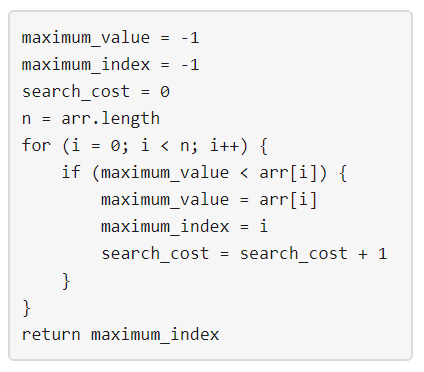# 生成数组

## 1420. 生成数组 (Hard)• `arr` 中有 `n` 个整数。
• `1 <= arr[i] <= m` 其中 `(0 <= i < n)`
• 将上面提到的算法应用于 `arr``search_cost` 的值等于 `k`

```输入：n = 2, m = 3, k = 1

```

```输入：n = 5, m = 2, k = 3

```

```输入：n = 9, m = 1, k = 1

```

```输入：n = 50, m = 100, k = 25

```

```输入：n = 37, m = 17, k = 7

```

• `1 <= n <= 50`
• `1 <= m <= 100`
• `0 <= k <= n`

[动态规划]